• 本篇博客主要讲的是如何将自己的图片数据集进行处理，然后搭建神经网络结构，训练数据，保存和加载模型，测试等过程
        为了学tensorflow，网上教程看了不少，大部分是利用mnist数据集，但是大部分都是利用已经处理好的非图片形式进行训练的；而且很多人都说不要自己造轮子，直接跑别人的代码，然后修改和学习，不过我觉得其实从数据集，到训练，到测试写下来，也有不少收获的。
本篇博客主要讲的是如何将自己的图片数据集进行处理，然后搭建神经网络结构，训练数据，保存和加载模型，测试等过程，其中利用mnist图片数据集(mnist)，代码(Github)。下面我分步讲解一下，跟大家一起学习。参考博客附后。
如前文所述，现在的入门教程基本是跑mnist代码，但是数据集是处理后的，那么如何处理自己的图像数据集？首先要将图片数据集制作成tfrecords格式的数据。简单的说，tfrecords是一种tensorflow方便快速读取数据的一种二进制文件，适用于大量数据的处理。下面的代码将说明如何将图像制作成tfrecords文件，该mnist图片数据集大小为(28,28,3)。

# current work dir
cwd = os.getcwd()

# data to int64List
def _int64_feature(value):
return tf.train.Feature(int64_list = tf.train.Int64List(value=[value]))
# data to floatlist
def _float_feature(value):
return tf.train.Feature(float_list = tf.train.FloatList(value=[value]))
# data to byteslist
def _bytes_feature(value):
return tf.train.Feature(bytes_list = tf.train.BytesList(value=[value]))

# convert image data to tfrecords
def generate_tfrecords(data_dir,filepath):
# gen a tfrecords object write
write = tf.python_io.TFRecordWriter(filepath)
#print cwd
for index,name in enumerate(num_classes):
#print class_path
file_dir = data_dir + name + '/'
for img_name in os.listdir(file_dir):
img_path = file_dir + img_name
print img_path
img_raw = img.tobytes()
example = tf.train.Example(features=tf.train.Features(feature={
'label':_int64_feature(index),
'img_raw':_bytes_feature(img_raw)}))
# convert example to binary string
write.write(example.SerializeToString())
write.close()

tf.python_io.TFRecordWriter返回一个writer对象用于将data_dir制作后的数据存入filepath中保存，该文件就是tfrecords文件。另外，tf.train.Example将数据处理成key-value(在这里就是标签-图像)的格式返回一个example对象。最后writer将数据写到filepath中，关闭writer，就完成了图片数据到二进制文件的制作过程。        制作完成之后，在神经网络中如何读取和解析呢？如下代码

# read and decode tfrecord
# produce file deque
filename_deque = tf.train.string_input_producer([filename])
# decode into fixed form
features = tf.parse_single_example(serialized_example,features={
'label':tf.FixedLenFeature([],tf.int64),
'img_raw':tf.FixedLenFeature([],tf.string)})

label = tf.cast(features['label'],tf.int32)
img = tf.decode_raw(features['img_raw'],tf.uint8)
img = tf.reshape(img,[28,28,3])
img = tf.cast(img,tf.float32)/255.-0.5
return label,img
其中，tf.train.string_input_producer([filename])是将filename的文件内容制作成一个队列，然后tf.parse_single_example按照固定的格式将内容解析出来，稍加处理即可得到label和img，当然[filename]中可以有很多file，因为当图片数据太大时可能会将数据分成好几个部分分别制作tfrecords进行存储和读取。
然后搭建神经网络，这里就搭建一个简单点的，如下

# create network
class network(object):
# define parameters w and b
def __init__(self):
with tf.variable_scope("Weight"):
self.weights={
'conv1':tf.get_variable('conv1',[5,5,3,32],initializer=tf.contrib.layers.xavier_initializer_conv2d()),
'conv2':tf.get_variable('conv2',[5,5,32,64],initializer=tf.contrib.layers.xavier_initializer_conv2d()),
'fc1'  :tf.get_variable('fc1',  [7*7*64,1024],initializer=tf.contrib.layers.xavier_initializer()),
'fc2'  :tf.get_variable('fc2',  [1024,10],     initializer=tf.contrib.layers.xavier_initializer()),}
with tf.variable_scope("biases"):
self.biases={
'conv1':tf.get_variable('conv1',[32,],initializer=tf.constant_initializer(value=0.0,dtype=tf.float32)),
'conv2':tf.get_variable('conv2',[64,],initializer=tf.constant_initializer(value=0.0,dtype=tf.float32)),
'fc1'  :tf.get_variable('fc1',  [1024,],initializer=tf.constant_initializer(value=0.0,dtype=tf.float32)),
'fc2'  :tf.get_variable('fc2',  [10,] ,initializer=tf.constant_initializer(value=0.0,dtype=tf.float32)),}

# define model
def model(self,img):
relu1 = tf.nn.relu(conv1)

relu2 = tf.nn.relu(conv2)

flatten = tf.reshape(pool2,[-1,self.weights['fc1'].get_shape().as_list()])

drop1 = tf.nn.dropout(flatten,0.8)
fc1   = tf.matmul(drop1,self.weights['fc1']) + self.biases['fc1']
fc_relu1 = tf.nn.relu(fc1)
fc2   = tf.matmul(fc_relu1,self.weights['fc2'])+self.biases['fc2']

return fc2

# define model test
def test(self,img):
img = tf.reshape(img,shape=[-1,28,28,3])

relu1 = tf.nn.relu(conv1)

relu2 = tf.nn.relu(conv2)

flatten = tf.reshape(pool2,[-1,self.weights['fc1'].get_shape().as_list()])

drop1 = tf.nn.dropout(flatten,1)
fc1   = tf.matmul(drop1,self.weights['fc1']) + self.biases['fc1']
fc_relu1 = tf.nn.relu(fc1)
fc2   = tf.matmul(fc_relu1,self.weights['fc2'])+self.biases['fc2']

return fc2

#loss
def softmax_loss(self,predicts,labels):
predicts = tf.nn.softmax(predicts)
labels   = tf.one_hot(labels,len(num_classes))
loss = -tf.reduce_mean(labels*tf.log(predicts))
self.cost = loss
return self.cost

# optimizer
def optimizer(self,loss,lr=0.001):
return train_optimizer
tf.contrib.layers.xavier_initializer_conv2d()是对参数进行初始化的，据某位童鞋的博客说，当激活函数是sigmoid或tanh时，这个初始化方法比较好，但是当激活函数是relu时，使用tf.contrib.layers.variance_scaling_initializer比较好，具体我没有尝试过，大家可以试一试，另外tf.contrib.layers.xavier_initializer()也是一种权值初始化方式。而在神经网络中，权值的初始化非常重要，可以按照某种特定的分布来初始化，以后可以尝试使用其他初始化方式从而加快收敛速度和准确率。
dropout层是一种解决过拟合的方法，它是在训练的过程中对网络中的某些神经单元按照一定概率屏蔽，此时的概率选择为0.8，但是在测试时可以不用dropout层，或者将概率设置为1，即使用所有的神经单元，这样应该能提高正确率。
模型搭建完毕就可以开始训练了，代码如下

def train():

net = network()
predicts = net.model(img_batch)
loss = net.softmax_loss(predicts,label_batch)
opti = net.optimizer(loss)
tf.summary.scalar('cost fuction',loss)
merged_summary_op = tf.summary.merge_all()

train_correct = tf.equal(tf.cast(tf.argmax(predicts,1),tf.int32),label_batch)
train_accuracy = tf.reduce_mean(tf.cast(train_correct,tf.float32))

#evaluate
test_out = net.test(test_img_batch)

test_correct = tf.equal(tf.cast(tf.argmax(test_out,1),tf.int32),test_label_batch)
test_accuracy = tf.reduce_mean(tf.cast(test_correct,tf.float32))

# init varibels
init = tf.global_variables_initializer()
with tf.Session() as sess:
sess.run(init)
coord = tf.train.Coordinator()
summary_writer = tf.summary.FileWriter('log',sess.graph)
# run deque
path =cwd+'/'+'model/model.ckpt'
#if os.path.exists(path):
try:
print "try to reload model ......"
tf.train.Saver(max_to_keep=None).restore(sess,path)
except:
finally:
print 'training .......'
for i in range(1,epoch+1):
#val,l= sess.run([img_batch,label_batch])
if i%50 ==0:
loss_np,_,label_np,img_np,predict_np = sess.run([loss,opti,label_batch,img_batch,predicts])
tr_accuracy_np = sess.run([train_accuracy])
print i,' epoch loss :',loss_np,'    train accuracy: ', tr_accuracy_np
if i%200==0:
summary_str,_l,_o = sess.run([merged_summary_op,loss,opti])
te_accuracy = sess.run([test_accuracy])
print 'test accuracy: ', te_accuracy
if i%1000==0:
tf.train.Saver(max_to_keep=None).save(sess,os.path.join('model','model.ckpt'))
# somethind happend that the thread should stop
coord.request_stop()
# wait for all threads should stop and then stop
coord.join(threads)

tf.summary.scalar('cost fuction',loss)
merged_summary_op = tf.summary.merge_all()
summary_writer = tf.summary.FileWriter('log',sess.graph)
summary_str,_l,_o = sess.run([merged_summary_op,loss,opti])


以上几行代码的搭配是将loss的变化存储在log文件中，当训练完成之后使用以下命令进行图形化展示，有些情况下直接使用tensorboard命令不一定管用，此时可以找到tensorboard.py目录，使用第二种命令进行展示.最后打开浏览器，访问终端提示的网址即可。
tensorboard --logdir=log
python 目录/tensorboard.py --logdir=log
关于evaluate部分，在训练过程中可以使用一部分数据集来验证模型的准确率，本程序将验证集合测试集视为相同。           coord = tf.train.Coordinator()#创建一个协调器，用于管理线程，发生错误时及时关闭线程          threads = tf.train.start_queue_runners(sess=sess,coord=coord)#各个线程开始读取数据，这一句如果没有，整个网络将被挂起          coord.request_stop()#某个线程数据读取完或发生错误请求停止          coord.join(threads)#所有线程都请求停止后关闭线程          以上几行代码的搭配是线程的开启和关闭过程，后面两句如果不存在，当读取过程出现某些错误(如Outofrange)时，程序将不会正常关闭等，详细情况大家可以查阅一下其他资料。            tf.train.Saver(max_to_keep=None).save(sess,os.path.join('model','model.ckpt'))           tf.train.Saver(max_to_keep=None).restore(sess,path)           以上两句是模型的保存和恢复，max_to_keep=None这个参数是保存最新的或者加载最新的模型。            accuracy_np = sess.run([accuracy])           以上是关于输出，如果希望得到某个输出A，那么只要使用A_out = sess.run([A])即可   参考博客：1. http://blog.csdn.net/hjimce/article/details/51899683
展开全文python mnist图片 Linux 深度学习
• 使用Tensorflow创建自己的数据集，并训练 自学入坑，尝试着写博客记录自己的学习过程，希望对其他人也有帮助 介绍环境 win10 + pycharm 介绍背景 小弟是某高校农业电气自动化学生，导师要求用卷积神经网络对...
使用Tensorflow创建自己的数据集，并训练
介绍环境
win10 + pycharm + CPU
介绍背景
要求用卷积神经网络对不同水分的玉米进行分类（最后的目标是实现回归，以后研究），神经网络虽然是科研神器，但是在工业上的应用效果远远不如实验室中的好。我们找到的教程无非是mnist，表情识别，等官方的数据集。对于一个小白来说虽然上手容易，但是收获这得有限。这篇博客我希望把每个知识都讲到尽量的通俗易懂，希望这篇处女作可以给小白指导。文中部分代码参考了ywx1832990，在此感谢。受限于水平，有讲解错误的地方，也欢迎留言探讨。
话不多说 直接上代码
step1：建立两个TFrecords
# pycharm中此模块名为genertateds.py

import os
import tensorflow as tf
from PIL import Image
# 源数据地址
cwd = r'C:\Users\pc\Desktop\orig_picture'
# 生成record路径及文件名
train_record_path =r"C:\Users\pc\Desktop\outputdata\train.tfrecords"
test_record_path =r"C:\Users\pc\Desktop\outputdata\test.tfrecords"
# 分类
classes = {'11.8','13','14.8','16.5','18','20.6','22.8','26.1','28.7','30.6'}

def _byteslist(value):
"""二进制属性"""
return tf.train.Feature(bytes_list = tf.train.BytesList(value = [value]))

def _int64list(value):
"""整数属性"""
return tf.train.Feature(int64_list = tf.train.Int64List(value = [value]))

def create_train_record():
"""创建训练集tfrecord"""
writer = tf.python_io.TFRecordWriter(train_record_path)     # 创建一个writer
NUM = 1                                     # 显示创建过程（计数）
for index, name in enumerate(classes):
class_path = cwd + "/" + name + '/'
l = int(len(os.listdir(class_path)) * 0.7)      # 取前70%创建训练集
for img_name in os.listdir(class_path)[:l]:
img_path = class_path + img_name
img = Image.open(img_path)
img = img.resize((128, 128))                # resize图片大小
img_raw = img.tobytes()                     # 将图片转化为原生bytes
example = tf.train.Example(                 # 封装到Example中
features=tf.train.Features(feature={
"label":_int64list(index),          # label必须为整数类型属性
'img_raw':_byteslist(img_raw)       # 图片必须为二进制属性
}))
writer.write(example.SerializeToString())
print('Creating train record in ',NUM)
NUM += 1
writer.close()                                      # 关闭writer
print("Create train_record successful!")

def create_test_record():
"""创建测试tfrecord"""
writer = tf.python_io.TFRecordWriter(test_record_path)
NUM = 1
for index, name in enumerate(classes):
class_path = cwd + '/' + name + '/'
l = int(len(os.listdir(class_path)) * 0.7)
for img_name in os.listdir(class_path)[l:]:     # 剩余30%作为测试集
img_path = class_path + img_name
img = Image.open(img_path)
img = img.resize((128, 128))
img_raw = img.tobytes()  # 将图片转化为原生bytes
# print(index,img_raw)
example = tf.train.Example(
features=tf.train.Features(feature={
"label":_int64list(index),
'img_raw':_byteslist(img_raw)
}))
writer.write(example.SerializeToString())
print('Creating test record in ',NUM)
NUM += 1
writer.close()
print("Create test_record successful!")

"""读取tfrecord"""
filename_queue = tf.train.string_input_producer([filename])     # 创建文件队列
features = tf.parse_single_example(
serialized_example,
features={
'label': tf.FixedLenFeature([], tf.int64),
'img_raw': tf.FixedLenFeature([], tf.string)
}
)
label = features['label']
img = features['img_raw']
img = tf.decode_raw(img, tf.uint8)
img = tf.reshape(img, [128, 128, 3])
img = tf.cast(img, tf.float32) * (1. / 255) - 0.5       # 归一化
label = tf.cast(label, tf.int32)
return img, label

def get_batch_record(filename,batch_size):
"""获取batch"""
image_batch,label_batch = tf.train.shuffle_batch([image,label],         # 随机抽取batch size个image、label
batch_size=batch_size,
capacity=2000,
min_after_dequeue=1000)
return image_batch,label_batch

def main():
create_train_record()
create_test_record()
if __name__ == '__main__':
main()

这里值得一提的是 from PIL import Image 在jupyter中某次更新后，会出现无法使用的现象。建议使用jupyter的朋友不要更新。如果更新了可以卸载，重新安装之前的版本
注意 windows下 cwd = r’C:\Users\pc\Desktop\orig_picture’的执行可能会产生一些操作系统层面的格式错误，在下面必须严格遵守 class_path = cwd + “/” + name + ‘/’ 这种格式，否则会出现格式错误。
train_record_path =r"C:\Users\pc\Desktop\outputdata\train.tfrecords" test_record_path =r"C:\Users\pc\Desktop\outputdata\test.tfrecords" 这两句代码的意思是定义了两个路径，因为这个模块的作用就是创建TFrecord（一种Tensorflow中管理数据的格式，只要想使用Tensorflow，就需要用TFrecord。TFRecord内部使用的是二进制编码，它可以很好的把数据一次性通过一个二进制文件读取进来，而不是一张图片一张图片的读取，节省了时间，增加了效率。）所以需要告诉计算机一个保存的路径
class中不同的数字 classes = {‘11.8’,‘13’,‘14.8’,‘16.5’,‘18’,‘20.6’,‘22.8’,‘26.1’,‘28.7’,‘30.6’} 就是不同的水分
writer = tf.python_io.TFRecordWriter() 这一部分是一个TFRecord的生成器，一般伴随着writer.write(),使用完之后需要关闭生成器，即：writer.close()
tf.train.Example：可以理解为一个包含了Features的内存块，并通过feature将图片的二进制数据和label进行统一封装（把数据和标签统一存储）， 然后将example转化为一种字符串的形式， 使用tf.python_io.TFRecordWriter() 写入到TFRecords文件中。
step2：配置图片的参数+定义前向传播过程
#这个模块在pycharm中的名字是forward.py

import tensorflow as tf
# 配置参数
# 图片size
IMAGE_SIZE = 128
NUM_CHANNELS = 3
NUM_LABELS = 10
# 第一层卷积层的尺寸和深度
CONV1_DEEP = 64
CONV1_SIZE = 5
# 第二层卷积层的尺寸和深度
CONV2_DEEP = 128
CONV2_SIZE = 5
# 全连接层的节点个数
FC_SIZE = 10
def get_Weight(shape,regularizer_rate = None):     # 定义weight如需正则化需传入zhengzehualv默认值为None
Weight = tf.Variable(tf.truncated_normal(shape=shape,stddev=0.1),dtype=tf.float32)    # tensorflow API推荐随机初始化

if regularizer_rate != None:
regularizer = tf.contrib.layers.l2_regularizer(regularizer_rate)

return Weight

def get_biase(shape):       # 定义biase
biase = tf.Variable(tf.constant(value=0.1,shape=shape),dtype=tf.float32)    # tensorflow API推荐初始化0.1
return biase

def create_conv2d(x,w):     # 定义卷积层
return conv2d

def max_pooling(x):         # 定义最大值池化
return pool

def create_fc(x,w,b):       # 定义全连接层
fc = tf.matmul(x,w) + b
return fc

# 定义前向传播的过程
# 这里添加了一个新的参数train，用于区分训练过程和测试过程。

def inference(input_tensor, train, regularizer_rate):
with tf.variable_scope('layer1-conv1'):
conv1_Weights = get_Weight([CONV1_SIZE,CONV1_SIZE,NUM_CHANNELS,CONV1_DEEP])     # 5*5*64
conv1_baises = get_biase([CONV1_DEEP])
conv1 = tf.nn.relu(conv1)       # 使用ReLu激活函数

with tf.name_scope('layer2-pool1'):        # 64*64*64
pool1 = max_pooling(conv1)

with tf.variable_scope('layer3-conv2'):
conv2_Weights = get_Weight([CONV2_SIZE,CONV2_SIZE,CONV1_DEEP,CONV2_DEEP])       # 5*5*128
conv2_biases = get_biase([CONV2_DEEP])
conv2 = tf.nn.relu(conv2)

with tf.name_scope('layer4-pool2'):         # 32*32*128
pool2 = max_pooling(conv2)

pool_shape = pool2.get_shape().as_list()
# pool_shape为[batch_size,32,32,128]
# 计算将矩阵拉直成向量之后的长度，这个长度就是矩阵长度及深度的乘积。
nodes = pool_shape * pool_shape * pool_shape
# 通过tf.reshape函数将第四层的输出变成一个batch的向量
reshaped = tf.reshape(pool2, [pool_shape, nodes])

# 声明第五层全连接层的变量并实现前向传播过程
with tf.variable_scope('layer5-fc1'):
fc1_Weights = get_Weight([nodes,FC_SIZE],regularizer_rate)
fc1_biases = get_biase([FC_SIZE])
fc1 = tf.nn.relu(create_fc(reshaped,fc1_Weights,fc1_biases))
# 训练过程添加dropout防止过拟合
if train:
fc1 = tf.nn.dropout(fc1, 0.5)

# 声明第六层全连接层的变量并实现前向传播过程
with tf.variable_scope('layer6-fc2'):
fc2_Weights = get_Weight([FC_SIZE,NUM_LABELS],regularizer_rate)
fc2_biases = get_biase([NUM_LABELS])
logit = create_fc(fc1,fc2_Weights,fc2_biases)
# fc2 = tf.nn.relu(fc2)     需要softmax层时使用激活函数

return logit

在写代码的过程中，我们应该把需要配置的参数在开头声明出来（一边写，写到哪个参数，就返回开头定义变量）
IMAGE_SIZE = 128 呼应了上一个模块中的reshape NUM_CHANNELS = 3 这里涉及到图像的知识，简单来说，设置为3就是彩色图片，设置为1就是灰度图 NUM_LABELS = 10 就是你所提前设置好的标签数（即需要分成多少个类）
CONV1_DEEP = 64 这里的conv卷积层，其实就是我们所说的filter。这里深度deep的意思就是一次性使用多少个filter （就是过滤器的个数，使用几个不同的过滤器，就是确定了深度是几） CONV1_SIZE = 5 确定卷积（filter）的size，即使用55的"观察窗口" 这样我们第一层的信息量就变成了了55*64
第二层卷积层同理（不懂的小伙伴可以留言）
FC_SIZE = 10 定义全连接层的个数，全连接层的一般是两层，最后一层全连接层就是输出层。全连接层的作用就是特征的加权，最后10个类的所有权值加在一起应该是等于1的。
def get_Weight 这里定义的是后面需要的权重值。 tf.truncated_normal(shape, mean, stddev) :shape表示生成张量的维度（这一部分后面用到了，注意一下），mean是均值，stddev是标准差。这个函数产生正太分布，均值和标准差自己设定。 regularizer_rate的意思是选择一种正则化方式，没有指定的话默认使用l2正则化。l2正则化简单理解就是可以去掉权重值中的超过两次幂的高此项，个人理解是防止过拟合用的。
def get_biase(shape): 定义一个偏置 tf.constant用来定义一个常量，这里的value一般初始化成一个常数或者一个数组。这里初始化成0.1是没有为什么的，不一定非要使用0.1。shape参数和卷积层的深度有关（即，和使用的filter数量有关，不能像0.1一样写死了，是需要变化的） tf.Variable(initializer,name)用来初始化一个变量。参数initializer是初始化参数，name是可自定义的变量名称
def max_pooling(x) 池化层的表面形式来看，十分类似于之前的卷积层。但是二者做的事情是不一样的。池化层的作用是为了避免无关的信息过多，干扰结果，或者影响计算速度所进行的一种降维，是一种特征工程。注意：池化层的输入就是卷积层的输出，这里隐含了一个条件，就是池化层必须在卷积层之后。 tf.nn.max_pool(value, ksize, strides, padding, name=None)中 第一个参数value是最需要注意的，这里的value输入的是feature map（在卷积层中，数据是以一维或者三维的形式存在的。RGB图片可以理解为3个二维图片叠在一起，其中每一个称为一个feature map。而灰度图就仅仅只存在一个feature map） ksize是池化窗口的大小，取一个四维向量，一般是[1, 1, 1, 1]或者[1, 2, 2, 1]。如果在这里你还在纠结为什么是[1, 1, 1, 1]或者[1, 2, 2, 1]，大可不必。在学习的初期，我们不应该被这种细枝末节的细节去分散走我们大多的精力，耗散我们的耐心，降低我们的成就感。你只需要知道，大家都这么用就好。 strides和padding参数的设置同上（没明白的请留言）
def create_fc(x,w,b): 定义一个全连接层，全连接层做的事情，前面已经有提过这里不做赘述，还希望深入了解全连接层作用的同学可以自行查找一些资料
def inference(input_tensor, train, regularizer_rate): 定义一个函数，构造神经网络：神经网络的结构为：输入→卷积层→池化层→卷积层→池化→全连接→全连接。 train这个参数在后面坐了一次if判断，关系到是否要进行dropout dropout可以理解为在防止过拟合。他的具体过程简要来说，就是不一次性给机器看到所有的数据，每次训练都随机较少一部分数据。 with tf.name_scope的意思就是方便你看代码的，类似于命名空间，不理解可以不看这部分，照着写就可以了。无关痛痒。
在代码运行的中间部分位置使用了一种激活函数–relu。relu函数可以理解为一种可以让机器学习到非线性特征的激活函数。因为我们之前做的无非是矩阵运算，这些都是属于线性运算（要不为啥是线性代数呢…）然而真是的世界中，事物往往都不是线性存在的，加入relu之后我们可以让机器学到这种非线性的特征
step3：定义反向传播网络
如果说之前我们在做的的是从前往后推导，模拟的是人类神经元的思维过程。那么反向传播网络在我看来，就已经超出了人类生物模型的范围，而变成了一种数学上的推导。可以说，在从前往后推导中我们已经建立一种可以用的模型，但是这个模型可以说是“惨不忍睹”，准确率低的令人大致，loss高得不忍直视。我们的前辈想到一种方法，提高模型的准确率。就是所谓的反向传播。通俗解释就是，基于现在的准确率，反向优化建模中所有的参数，再用经过优化的参数，重复建模的过程，再得到一个准确率，一般来说，经过一次优化的参数会提高模型的准确率。那么不断重复之前的过程，重复n多次，在不出现过拟合的前提下，我们最终就可以得到一个很优秀的模型。下面上代码
#这个模块命名为backward.py
#这里需要注意，import genertateds 和 import forward 导入的是刚才定义好的模块，这里体现出了python的继承特点。对python理解不深的同学需要花时间理解一下什么是继承

import tensorflow as tf
import forward
import os
import genertateds
# 定义神经网络相关参数
BACTH_SIZE = 100
LEARNING_RATE_BASE=0.1
LEARNING_RATE_DECAY = 0.99
REGULARAZTION_RATE = 0.0001
TRAINING_STEPS = 150
MOVING_AVERAGE_DECAY = 0.99
train_num_examples = 500
# 模型保存的路径和文件名
MODEL_SAVE_PATH = "LeNet5_model_of_corn1234/"
MODEL_NAME = "LeNet5_model_of_corn1234"
# 定义训练过程
def train():
# 定义输入输出的placeholder
x = tf.placeholder(tf.float32, [
BACTH_SIZE,
forward.IMAGE_SIZE,
forward.IMAGE_SIZE,
forward.NUM_CHANNELS])
y_ = tf.placeholder(tf.int32, [None], name='y-input')       # label为int类型

y = forward.inference(x,True,REGULARAZTION_RATE)            # 训练过程需要使用正则化

global_step = tf.Variable(0, trainable=False)               # 记录step、不可训练的变量

# 定义滑动平均类
variable_average = tf.train.ExponentialMovingAverage(MOVING_AVERAGE_DECAY, global_step)
variable_average_op = variable_average.apply(tf.trainable_variables())
# 定义损失函数
# cross_entropy_mean = tf.reduce_mean(tf.square(y - y_))    # 使用softmax层时的loss函数

cross_entropy = tf.nn.sparse_softmax_cross_entropy_with_logits(logits=y, labels=y_)
cross_entropy_mean = tf.reduce_mean(cross_entropy)
# 定义指数衰减学习率
learning_rate = tf.train.exponential_decay(LEARNING_RATE_BASE,
global_step,
train_num_examples/BACTH_SIZE,
LEARNING_RATE_DECAY,
staircase=True)
# 控制计算流程（自己这么理解的...）
with tf.control_dependencies([train_step, variable_average_op]):
train_op = tf.no_op(name='train')

# 初始化TensorFlow持久化类
saver = tf.train.Saver()
# 读取训练集
image_batch,label_batch = genertateds.get_batch_record(genertateds.train_record_path,100)

with tf.Session() as sess:
# 初始化所有变量
init_op = tf.global_variables_initializer()
sess.run(init_op)
# 断点检查
ckpt = tf.train.get_checkpoint_state(MODEL_SAVE_PATH)
# 有checkpoint的话继续上一次的训练
if ckpt and ckpt.model_checkpoint_path:
saver.restore(sess,ckpt.model_checkpoint_path)
# 创建线程
coord = tf.train.Coordinator()
# 开始训练
for i in range(TRAINING_STEPS):
xs, ys = sess.run([image_batch,label_batch])
_, loss_value, step = sess.run([train_op, loss, global_step],
feed_dict={x:xs,y_:ys})
# 每20轮保存一次模型
if i % 20 == 0:
# 输出当前的训练情况
print("After %d training step(s),loss on training batch is %g." % (step, loss_value))
# 保存当前模型
saver.save(
sess, os.path.join(MODEL_SAVE_PATH, MODEL_NAME), global_step=global_step)
# 关闭线程
coord.request_stop()
def main():
train()

if __name__ == '__main__':
main()

BACTH_SIZE = 100 这个参数是批尺寸。这是在说，每次拿全部数据中的的一部分过来训练。减少单次的数量，减少时间。如果本身的数据不是很多的话，完全可以直接把全部数据拿过来训练，不需要BACTH_SIZE 这个参数。其实我本身的数据也不是很多，设置这个参数是为了通用性。需要注意的是BACTH_SIZE的值不能太大，也不能太小。太大内存吃不消，也打不到分批的效果。太小可能会出现由于每次取的batch差异性太大，从而使梯度互相抵消，造成结果不收敛。
LEARNING_RATE_BASE=0.1 （学习率的基值） LEARNING_RATE_DECAY = 0.99 （一般设为接近于1的值） 这两个参数可以合在一起说，因为一般而言，在训练模型的初期，学习率是比较大的，随着训练的步数越来越多，再使用很大的学习率会出现无法收敛的现象（步子太大，本来很小的步子就可以达到最优点，可是直接迈过了最优点。所以经验而谈，最开始可以大步慢走，越深入，越接近最优点，就应该使用小步快跑的方式）这两个参数在后面就是tensorflow中一个调整学习率的API需要的参数。所谓的LEARNING_RATE_BASE=0.1就是初始学习率，LEARNING_RATE_DECAY = 0.99就是学习率的衰减率
REGULARAZTION_RATE = 0.0001 这个参数可以参看前面我对forward.py的描述
TRAINING_STEPS = 150 这个很好理解，就是后面训练的步数
MOVING_AVERAGE_DECAY = 0.99 train_num_examples = 500 这两个参数我们暂时按下不表，后面马上会说到
MODEL_SAVE_PATH = “LeNet5_model_of_corn1234/” MODEL_NAME = “LeNet5_model_of_corn1234” 以上这两步看着不起眼，但是确实是整个系统中十分重要的一步，也是反向传播网络的灵魂。笔者开始也是在这个位置没有深入理解，导致后面的思维偏离正规。我们需要再次确认，我们现在定义的这个神经网络是在干什么？我希望看到这篇文章的人都可以先不要往后看。先思考5分钟。明白神经网络在干什么，这是对一个新手来说是非常非常重要的。
那么我们下面公布答案，最简单的话说，就是建模。
不管我们在神经网络中，把程序写的多么花哨，多么天花乱坠，神经网络做的事情也仅仅是建模。为什么在网上看到神经网络，最后输出的往往是“准确率”？ 难道不是应该对我们的输入进行分类，最后给出一个明确的结果吗？为什么会是一个所谓的“准确率”呢？
不是的，在我们这个构建神经网络的过程中，我们在做的是建模，后面的准确率的本质是，你的测试集在对你构建的模型进行评估，评估模型的准确率。在这里其实并不涉及到具体的分类。
那么具体的分类怎么做呢？答案是，把训练好的模型保存下来，然后再写另外一套别的程序（这部分，我自己还没有具体的理性认识，研究明白了后面会写新的博客）然后我们就要说道刚刚的两句代码了，这两句的代码其实就是在声明一个模型保存的路径和文件名，便于我们以后加载自己的模型，对新的输入进行评估。
def train():这个 模块整个就是在具体的表现训练的过程 x和 y_是两个占位符。所谓占位符，也是不需要太过纠结的细节问题，简单说，就是数据不会是上来一次性全都给你的，而是需要在不同的时间点，分批次给你的。也就是说，输入是在变化的，那么这样，我们在写程序的时候就不可以把程序的输入写死。定义占位符可以完美的解决问题，数据不断的被输入到占位符，程序每次向占位符要数据，然后占位符中的数据更新。
y = forward.inference(x,True,REGULARAZTION_RATE) 这里用了forward.py模块中的一个函数，不理解的同学大可以向上翻。 global_step = tf.Variable(0, trainable=False) 在这里定义了一个“不可训练的变量” ，这个不可训练的变量需要人为设置，并将该参数传入我们的模型。这个参数在后面马上可以用到。
variable_average = tf.train.ExponentialMovingAverage(MOVING_AVERAGE_DECAY, global_step) variable_average_op = variable_average.apply(tf.trainable_variables()) 这两句代码定义了一个滑动平均类，什么是滑动平均类呢？简而言之，它也是用来更新参数的一个函数，而这种更新参数的方式就是滑动平均，这仅仅是一个名字而已。这个函数初始化需要提供一个衰减速率（decay），用于控制模型的更新速度。MOVING_AVERAGE_DECAY就是这里的衰减速度。可以看到，第二不到吗，等式左边的参数是可以更新的变量，global不参与这里的更新，所以把它设置为不可更新。
cross_entropy = tf.nn.sparse_softmax_cross_entropy_with_logits(logits=y, labels=y_) cross_entropy_mean = tf.reduce_mean(cross_entropy) loss = cross_entropy_mean + tf.add_n(tf.get_collection(‘losses’)) 这一部分是在定义交叉熵损失函数，交叉熵softmax是在做什么事呢？其实softmax说白了就是在做回归处理。神经网络的本来输出的并不是概率，而是一些经过了复杂的加权，加偏置，非线性处理之后的一个值而已，如何才能让这样一个值变成我们理解的概率值？这就需要softmax。softmax=一部分/一个整体，最后输出的自然就是一个概率值了。（这里不写公式，想看的话网上到处都有） loss最后就等于经过交叉熵之后对张量取平均
learning_rate = tf.train.exponential_decay(LEARNING_RATE_BASE,global_step,train_num_examples/BACTH_SIZE, LEARNING_RATE_DECAY, staircase=True) 这里再定义指数衰减学习率，LEARNING_RATE_BASE是学习率基础值。global_step就是那个手动指定的不能训练的参数 train_num_examples/BACTH_SIZE是衰减的速度，即更新学习率的速度（注意区分，不是学习率本身的变化幅度，而是多长时间需要去变一次。即总训练样本/每次的feed的batch）LEARNING_RATE_DECAY前面提过，是学习率的衰减率（即学习率本身变化的幅度）staircase=True的意思就是梯度衰减，False为平滑衰减。这里借用一张图来解释，何为梯度衰减，何为平滑衰减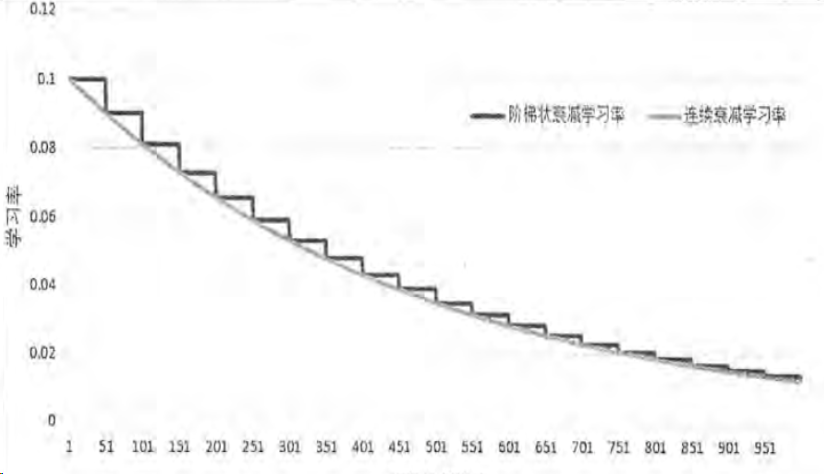with tf.control_dependencies([train_step, variable_average_op]): train_op = tf.no_op(name=‘train’) 这里是一个上下文管理器（我自己依旧是按照命名空间来理解的）这部分代码完全不需要背，可以理解为一个固定流程
saver = tf.train.Saver() image_batch,label_batch = genertateds.get_batch_record(genertateds.train_record_path,100) 这两步，对于有基础的人，应该比较好理解。无非是一个保存模型，一个用来读取训练集中的图片和标签。
with tf.Session() as sess: 这里面在做的事有：1.初始化所有变量（必须加，不要问为什么，加就好了。Tensorflow要求你必须加这两步，否则不work） 2.断点检查 这个意思是你可以在上次训练的模型基础上，接着训练，不需要每次都从头开始 3.创建线程 对于线程，进程，携程不理解的同学需要自行google，这里把这个知识点展开了将不现实（如果需要以后开新的文章介绍） 总而言之，就是你不能把所用东西一次性丢给计算机，而是需要建立一个线程，主线程执行你本应该执行的代码，子线程可以去分批次的拿数据，子线程拿过来一点，主线程就训练一点，边拿边训练。（就好像是本来只有一只手干活，这是手是主线程。主线程忙着的时候，子线程就可以去做一些辅助主线程的工作，来配合子线程） 4.训练，每10轮保存一次模型（随时可以中断，再开启时会自动从上次中断的位置来，生成ckpt文件）
step4：输出测试集的测试结果
#这个模块我命名为test.py
#导入之前的三个模块，分别是genertateds，forward，backward
import time
import forward
import backward
import genertateds
import tensorflow as tf

# 等待时间
TEST_INTERVAL_SECS = 5
# 总测试集样本数量
test_num_examples = 20
def test():
with tf.Graph().as_default() as g:
x = tf.placeholder(tf.float32,[test_num_examples,
forward.IMAGE_SIZE,
forward.IMAGE_SIZE,
forward.NUM_CHANNELS])
y_ = tf.placeholder(tf.int64,[None])
# 测试过程不需要正则化和dropout
y = forward.inference(x,False,None)
# 还原模型中的滑动平均
variable_average = tf.train.ExponentialMovingAverage(backward.MOVING_AVERAGE_DECAY)
variable_average_restore = variable_average.variables_to_restore()
saver = tf.train.Saver(variable_average_restore)
# 计算准确率
correct_prediction = tf.equal(tf.argmax(y,1),y_)
accuracy = tf.reduce_mean(tf.cast(correct_prediction,tf.float32))
image_batch,label_batch = genertateds.get_batch_record(genertateds.test_record_path,20)

while True:
with tf.Session() as sess:

ckpt = tf.train.get_checkpoint_state(backward.MODEL_SAVE_PATH)
if ckpt and ckpt.model_checkpoint_path:

coord = tf.train.Coordinator()

image, label = sess.run([image_batch, label_batch])

saver.restore(sess,ckpt.model_checkpoint_path)
# 从文件名称中读取第几次训练
global_step = ckpt.model_checkpoint_path.split('/')[-1].split('-')[-1]
accuracy_score = sess.run(accuracy,feed_dict={x:image,y_:label})

coord.request_stop()
print("After %s training step(s),test accuray = %g"%(global_step,accuracy_score))
else:
time.sleep(TEST_INTERVAL_SECS)

def main():
test()
if __name__ == '__main__':
main()


#等待时间 TEST_INTERVAL_SECS = 5 #总测试集样本数量 test_num_examples = 20 这里再声明两个变量，声明等待时间在后面会用到。time.sleep(TEST_INTERVAL_SECS)的意思是让程序执行到这里，休眠5秒钟，什么都不做。（这个时候cpu可以去处理其他的任何事，只是当前的程序需要休眠）。test_num_examples = 20是后面的占位符需要的参数
def test(): 这个函数里做了什么事呢？ 首先明确一点，模块名字是test，那么自然，这个函数做的就是验证模型的好坏。怎么评价模型的还坏？自然是对比着验证集来看模型准确率 x和y_是两个占位符，前面已经提过 y由于是在测试，没有正则化处理
variable_average = tf.train.ExponentialMovingAverage(backward.MOVING_AVERAGE_DECAY) variable_average_restore=variable_average.variables_to_restore() saver = tf.train.Saver(variable_average_restore) 这里做的之前也有讲过，不再赘述
tf.argmax(input,axis)用来返回返回每行或者每列最大值的索引 tf.equal是一个判等函数 结合起来就是在判断，最大索引值，和测试集中传进来的值是否一致
accuracy= tf.reduce_mean(tf.cast(correct_prediction,tf.float32)) image_batch,label_batch=genertateds.get_batch_record(genertateds.test_record_path,20)这两句代码的细节在上个模块中也提到了，忘记了不妨回看，加深印象
while True:循环 不断的从ckpt中找到训练的模型，用来提供给后面，进行test
到此 一个完整的使用数据集搭建神经网络的过程就完成了闭环。
展开全文tensorflow 机器学习 训练模型
• ## 神经网络学习 之 BP神经网络

万次阅读 多人点赞 2015-11-30 21:17:56
上一次我们讲了M-P模型，它实际上就是对单个神经元的一种建模，...本文具体来介绍一下一种非常常见的神经网络模型——反向传播(Back Propagation)神经网络。概述BP（Back Propagation）神经网络是1986年由Rumelhart和Mc
上一次我们讲了M-P模型，它实际上就是对单个神经元的一种建模，还不足以模拟人脑神经系统的功能。由这些人工神经元构建出来的网络，才能够具有学习、联想、记忆和模式识别的能力。BP网络就是一种简单的人工神经网络。 本文具体来介绍一下一种非常常见的神经网络模型——反向传播(Back Propagation)神经网络。
概述
BP（Back Propagation）神经网络是1986年由Rumelhart和McCelland为首的科研小组提出，参见他们发表在Nature上的论文 Learning representations by back-propagating errors 。
BP神经网络是一种按误差逆传播算法训练的多层前馈网络，是目前应用最广泛的神经网络模型之一。BP网络能学习和存贮大量的 输入-输出模式映射关系，而无需事前揭示描述这种映射关系的数学方程。它的学习规则是使用最速下降法，通过反向传播来不断 调整网络的权值和阈值，使网络的误差平方和最小。
BP算法的基本思想
上一次我们说到，多层感知器在如何获取隐层的权值的问题上遇到了瓶颈。既然我们无法直接得到隐层的权值，能否先通过输出层得到输出结果和期望输出的误差来间接调整隐层的权值呢？BP算法就是采用这样的思想设计出来的算法，它的基本思想是,学习过程由信号的正向传播与误差的反向传播两个过程组成。
正向传播时，输入样本从输入层传入,经各隐层逐层处理后,传向输出层。若输出层的实际输出与期望的输出(教师信号)不符,则转入误差的反向传播阶段。反向传播时，将输出以某种形式通过隐层向输入层逐层反传,并将误差分摊给各层的所有单元,从而获得各层单元的误差信号,此误差信号即作为修正各单元权值的依据。
这两个过程的具体流程会在后文介绍。
BP算法的信号流向图如下图所示BP网络特性分析——BP三要素
我们分析一个ANN时，通常都是从它的三要素入手，即 1)网络拓扑结构； 2)传递函数； 3)学习算法。每一个要素的特性加起来就决定了这个ANN的功能特性。所以，我们也从这三要素入手对BP网络的研究。
3.1 BP网络的拓扑结构
上一次已经说了，BP网络实际上就是多层感知器，因此它的拓扑结构和多层感知器的拓扑结构相同。由于单隐层（三层）感知器已经能够解决简单的非线性问题，因此应用最为普遍。三层感知器的拓扑结构如下图所示。 一个最简单的三层BP：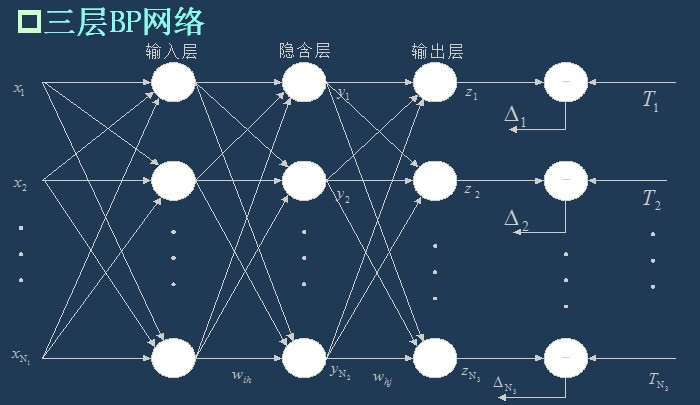###3.2 BP网络的传递函数 BP网络采用的传递函数是非线性变换函数——Sigmoid函数（又称S函数）。其特点是函数本身及其导数都是连续的，因而在处理上十分方便。为什么要选择这个函数，等下在介绍BP网络的学习算法的时候会进行进一步的介绍。 单极性S型函数曲线如下图所示。

f

(

x

)

=

1

1

+

e

−

x

f(x)={1\over 1+e^{-x}}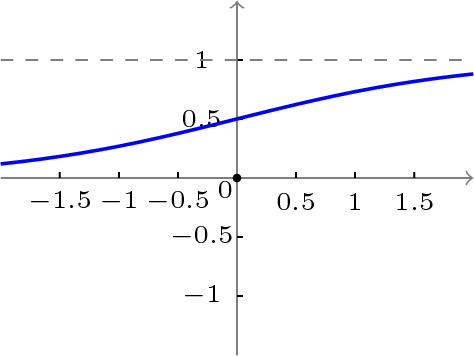双极性S型函数曲线如下图所示。

f

(

x

)

=

1

−

e

−

x

1

+

e

−

x

f(x)={1-e^{-x}\over 1+e^{-x}}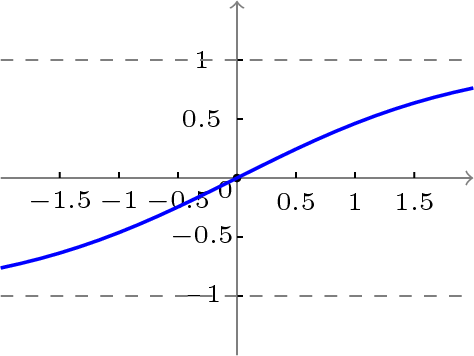3.3 BP网络的学习算法
BP网络的学习算法就是BP算法，又叫 δ 算法（在ANN的学习过程中我们会发现不少具有多个名称的术语）， 以三层感知器为例，当网络输出与期望输出不等时，存在输出误差 E ，定义如下

E

=

1

2

(

d

−

O

)

2

=

1

2

∑

κ

=

1

ℓ

(

d

k

−

o

k

)

2

E={1\over 2}(d−O)^2={1\over 2}∑_{κ=1}^ℓ(d_k−o_k)^2

将以上误差定义式展开至隐层，有

E

=

1

2

∑

κ

=

1

ℓ

[

d

κ

−

f

(

n

e

t

κ

)

]

2

=

1

2

∑

κ

=

1

ℓ

[

d

κ

−

f

(

∑

j

=

0

m

ω

j

κ

y

j

)

]

2

E={1\over 2}∑_{κ=1}^ℓ[d_κ−f(net_κ)]^2={1\over 2}∑_{κ=1}^ℓ[d_κ−f(∑_{j=0}^mω_{jκ}y_j)]^2

进一步展开至输入层，有

E

=

1

2

∑

κ

=

1

ℓ

d

κ

−

f

[

∑

j

=

0

m

ω

j

κ

f

(

n

e

t

j

)

]

2

=

1

2

∑

κ

=

1

ℓ

d

κ

−

f

[

∑

j

=

0

m

ω

j

κ

f

(

∑

j

=

0

n

υ

i

j

χ

i

)

]

2

E={1\over 2}∑_{κ=1}^ℓ{d_κ−f[∑_{j=0}^mω_{jκ}f(net_j)]}^2={1\over 2}∑_{κ=1}^ℓ{d_κ−f[∑_{j=0}^mω_{jκ}f(∑_{j=0}^nυ_{ij}χ_i)]}^2

由上式可以看出，网络输入误差是各层权值

ω

j

κ

ω_{jκ}

、

υ

i

j

υ_{ij}

的函数，因此调整权值可改变误差

E

E

。 显然，调整权值的原则是使误差不断减小，因此应使权值与误差的梯度下降成正比，即

Δ

ω

j

κ

=

−

η

∂

E

∂

ω

j

κ

j

=

0

,

1

,

2

,

…

,

m

;

κ

=

1

,

2

,

…

,

ℓ

Δω_{jκ}=−η{∂E\over ∂ω_{jκ}}j=0,1,2,…,m;κ=1,2,…,ℓ

Δ

υ

i

j

=

−

η

∂

E

∂

υ

i

j

i

=

0

,

1

,

2

,

…

,

n

;

j

=

1

,

2

,

…

,

m

Δυ_{ij}=−η{∂E∂\over υ_{ij}}i=0,1,2,…,n;j=1,2,…,m

对于一般多层感知器，设共有

h

h

个隐层，按前向顺序各隐层节点数分别记为

m

1

,

m

2

,

…

,

m

h

m_1,m_2,…,m_h

，各隐层输出分别记为

y

1

,

y

2

,

…

,

y

h

y_1,y_2,…,y_h

，各层权值矩阵分别记为

W

1

,

W

2

,

…

,

W

h

,

W

h

+

1

W_1,W_2,…,W_h,W_{h+1}

，则各层权值调整公式为
输出层

Δ

ω

j

κ

h

+

1

=

η

δ

h

+

1

κ

y

j

h

=

η

(

d

κ

−

o

κ

)

o

κ

(

1

−

o

κ

)

y

j

κ

(

j=0,1,2,

…

,mh;κ=1,2,

…

,ℓ

)

Δω^{h+1}_{jκ}=ηδ_{h+1}^κy^h_j=η(d_κ−o_κ)o_κ(1−o_κ)y^κ_j\tag{j=0,1,2,…,mh;κ=1,2,…,ℓ}

第

h

h

隐层

Δ

ω

i

j

h

=

η

δ

j

h

y

i

h

−

1

=

η

(

∑

κ

=

1

l

δ

κ

o

ω

j

κ

h

+

1

y

j

κ

(

1

−

y

j

k

a

p

p

a

)

y

i

h

−

1

(

i=0,1,2,

…

,m(h−1);j=1,2,

…

,

m

h

)

Δω^h_{ij}=ηδ^h_jy^h_i−1=η(∑^l_{κ=1}δ^o_κω^{h+1}_{jκ}y^κ_j(1−y^k_jappa)y^h_i−1\tag{i=0,1,2,…,m(h−1);j=1,2,…,$m_h$}

按以上规律逐层类推，则第一隐层权值调整公式

Δ

ω

p

q

1

=

η

δ

q

1

χ

p

=

η

(

∑

r

=

1

m

2

δ

r

2

ω

q

r

2

)

y

q

1

(

1

−

y

q

1

)

χ

p

(

p=0,1,2,

…

,n;j=1,2,

…

,

m

1

)

Δω^1_{pq}=ηδ^1_qχ_p=η(∑^{m_2}_{r=1}δ^2_rω^2_{qr})y^1_q(1−y^1_q)χ_p\tag{p=0,1,2,…,n;j=1,2,…,$m_1$}

容易看出，BP学习算法中，各层权值调整公式形式上都是一样的，均由3个因素决定，即：
学习率

η

η

本层输出的误差信号

δ

δ

本层输入信号

Y

Y

（或

X

X

）
其中输入层误差信号与网络的期望输出与实际输出之差有关，直接反应了输出误差，而各隐层的误差信号与前面各层的误差信号有关，是从输出层开始逐层反传过来的。
可以看出BP算法属于δ学习规则类，这类算法常被称为误差的梯度下降算法。δ学习规则可以看成是Widrow-Hoff(LMS)学习规则的一般化(generalize)情况。LMS学习规则与神经元采用的变换函数无关，因而不需要对变换函数求导，δ学习规则则没有这个性质，要求变换函数可导。这就是为什么我们前面采用Sigmoid函数的原因。
综上所述，BP三要素如下图所示。下面我们会介绍BP网络的学习训练的具体过程。
BP网络的训练分解
训练一个BP神经网络，实际上就是调整网络的权重和偏置这两个参数，BP神经网络的训练过程分两部分：
前向传输，逐层波浪式的传递输出值；逆向反馈，反向逐层调整权重和偏置； 我们先来看前向传输。
前向传输（Feed-Forward前向反馈）
在训练网络之前，我们需要随机初始化权重和偏置，对每一个权重取

[

−

1

,

1

]

[-1,1]

的一个随机实数，每一个偏置取

[

0

,

1

]

[0,1]

的一个随机实数，之后就开始进行前向传输。
神经网络的训练是由多趟迭代完成的，每一趟迭代都使用训练集的所有记录，而每一次训练网络只使用一条记录，抽象的描述如下：
while 终止条件未满足：
for record:dataset:
trainModel(record)

首先设置输入层的输出值，假设属性的个数为100，那我们就设置输入层的神经单元个数为100，输入层的结点

N

i

N_i

为记录第

i

i

维上的属性值

x

i

x_i

。对输入层的操作就这么简单，之后的每层就要复杂一些了，除输入层外，其他各层的输入值是上一层输入值按权重累加的结果值加上偏置，每个结点的输出值等该结点的输入值作变换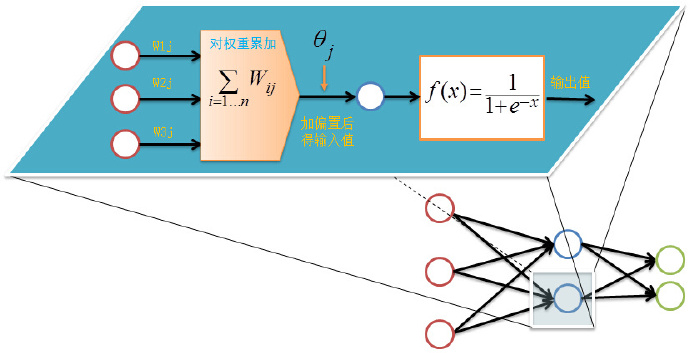前向传输的输出层的计算过程公式如下：

I

j

=

∑

i

=

1

ω

i

j

o

i

+

θ

j

I_j=\sum_{i=1}\omega_{ij}o_i+\theta_j

o

j

=

f

(

I

j

)

=

1

1

+

e

I

j

o_j=f(I_j)={1\over 1+e^{I_j}}

对隐藏层和输出层的每一个结点都按照如上图的方式计算输出值，就完成前向传播的过程，紧接着是进行逆向反馈。
逆向反馈（Backpropagation）
逆向反馈从最后一层即输出层开始，我们训练神经网络作分类的目的往往是希望最后一层的输出能够描述数据记录的类别，比如对于一个二分类的问题，我们常常用两个神经单元作为输出层，如果输出层的第一个神经单元的输出值比第二个神经单元大，我们认为这个数据记录属于第一类，否则属于第二类。
还记得我们第一次前向反馈时，整个网络的权重和偏置都是我们随机取，因此网络的输出肯定还不能描述记录的类别，因此需要调整网络的参数，即权重值和偏置值，而调整的依据就是网络的输出层的输出值与类别之间的差异，通过调整参数来缩小这个差异，这就是神经网络的优化目标。对于输出层：

E

j

=

O

j

(

1

−

O

j

)

(

T

j

−

O

j

)

E_j=O_j(1-O_j)(T_j-O_j)

其中

E

j

E_j

表示第

j

j

个结点的误差值，

O

j

O_j

表示第

j

j

个结点的输出值，

T

j

T_j

记录输出值，比如对于2分类问题，我们用01表示类标1,10表示类别2，如果一个记录属于类别1，那么其

T

1

=

0

T_1=0

，

T

2

=

1

T_2=1

。
中间的隐藏层并不直接与数据记录的类别打交道，而是通过下一层的所有结点误差按权重累加，计算公式如下：

E

j

=

O

j

(

1

−

O

j

)

∑

k

E

k

W

j

k

E_j=O_j(1-O_j)\sum_kE_kW_{jk}

其中

W

j

k

W_{jk}

表示当前层的结点

j

j

到下一层的结点

k

k

的权重值，

E

k

E_k

下一层的结点

k

k

的误差率。
计算完误差率后，就可以利用误差率对权重和偏置进行更新，首先看权重的更新：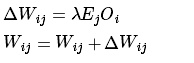其中

λ

λ

表示表示学习速率，取值为0到1，学习速率设置得大，训练收敛更快，但容易陷入局部最优解，学习速率设置得比较小的话，收敛速度较慢，但能一步步逼近全局最优解。
更新完权重后，还有最后一项参数需要更新，即偏置：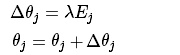至此，我们完成了一次神经网络的训练过程，通过不断的使用所有数据记录进行训练，从而得到一个分类模型。不断地迭代，不可能无休止的下去，总归有个终止条件。
训练终止条件
每一轮训练都使用数据集的所有记录，但什么时候停止，停止条件有下面两种：
设置最大迭代次数，比如使用数据集迭代100次后停止训练计算训练集在网络上的预测准确率，达到一定门限值后停止训练
BP网络运行的具体流程
网络结构
输入层有

n

n

个神经元，隐含层有

p

p

个神经元，输出层有

q

q

个神经元。
变量定义
输入变量：

x

=

(

x

1

,

x

2

,

…

,

x

n

)

x=(x_1,x_2,\dots,x_n)

隐含层输入变量：

h

i

=

(

h

i

1

,

h

i

2

,

…

,

h

i

p

)

hi=(hi_1,hi_2,\dots,hi_p)

隐含层输出变量：

h

o

=

(

h

o

1

,

h

o

2

,

…

,

h

o

p

)

ho=(ho_1,ho_2,\dots,ho_p)

输出层输入变量：

y

i

=

(

y

i

1

,

y

i

2

,

…

,

y

i

q

)

yi=(yi_1,yi_2,\dots,yi_q)

输出层输出变量：

y

o

=

(

y

o

1

,

y

o

2

,

…

,

y

o

q

)

yo=(yo_1,yo_2,\dots,yo_q)

期望输出向量：

d

o

=

(

d

1

,

d

2

,

…

,

d

q

)

d_o=(d_1,d_2,\dots,d_q)

输入层与中间层的连接权值：

w

i

h

w_{ih}

隐含层与输出层的连接权值：

w

h

o

w_{ho}

隐含层各神经元的阈值：

b

h

b_h

输出层各神经元的阈值：

b

o

b_o

样本数据个数：

k

=

1

,

2

,

…

,

m

k=1,2,\dots,m

激活函数：

f

(

⋅

)

f(\cdot)

误差函数：

e

=

1

2

∑

o

=

1

q

(

d

o

(

k

)

−

y

o

o

(

k

)

)

2

e={1\over 2}\sum_{o=1}^{q}(d_o(k)-yo_o(k))^2

###第一步：网络初始化 给各连接权值分别赋一个区间

(

−

1

,

1

)

(-1,1)

内的随机数，设定误差函数

e

e

，给定计算精度值

ε

\varepsilon

和最大学习次数

M

M

。 ###第二步：随机选取 随机选取第

k

k

个输入样本以及对应的期望输出

x

(

k

)

=

(

x

1

(

k

)

,

x

2

(

k

)

,

…

,

x

n

(

k

)

)

d

o

(

k

)

=

(

d

1

(

k

)

,

d

2

(

k

)

,

…

,

d

q

(

k

)

)

x(k)=(x_1(k),x_2(k),\dots,x_n(k))\\ d_o(k)=(d_1(k),d_2(k),\dots,d_q(k))

###第三部：隐含层计算 计算隐含层各神经元的输入和输出

h

i

h

(

k

)

=

∑

i

=

1

n

w

i

h

x

i

(

k

)

−

b

h

(

h

=

1

,

2

,

…

,

p

)

hi_h(k)=\sum_{i=1}^nw_{ih}x_i(k)-b_h \tag{$h=1,2,\dots,p$}

h

i

h

(

k

)

=

f

(

h

i

h

(

k

)

)

(

h

=

1

,

2

,

…

,

p

)

hi_h(k)=f(hi_h(k)) \tag{$h=1,2,\dots,p$}

y

i

o

(

k

)

=

∑

h

=

1

p

w

h

o

h

o

h

(

k

)

−

b

o

(

o=1,2,

…

,q

)

yi_o(k)=\sum_{h=1}^pw_{ho}ho_h(k)-b_o \tag{o=1,2,$\dots$,q}

y

o

o

(

k

)

=

f

(

y

i

o

(

k

)

)

(

o=1,2,

…

,q

)

yo_o(k)=f(yi_o(k))\tag{o=1,2,$\dots$,q}

第四步：求偏导数
利用网络期望输出和实际输出，计算误差函数对输出层的各神经元的偏导数

δ

o

(

k

)

\delta_o(k)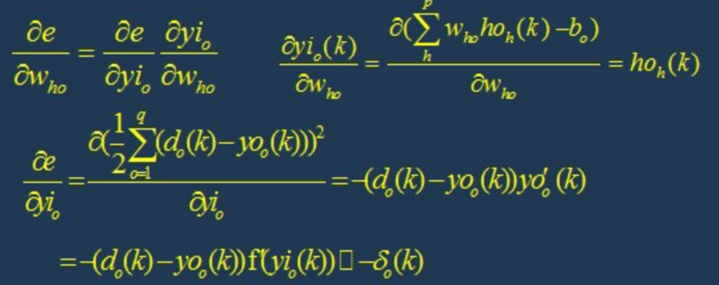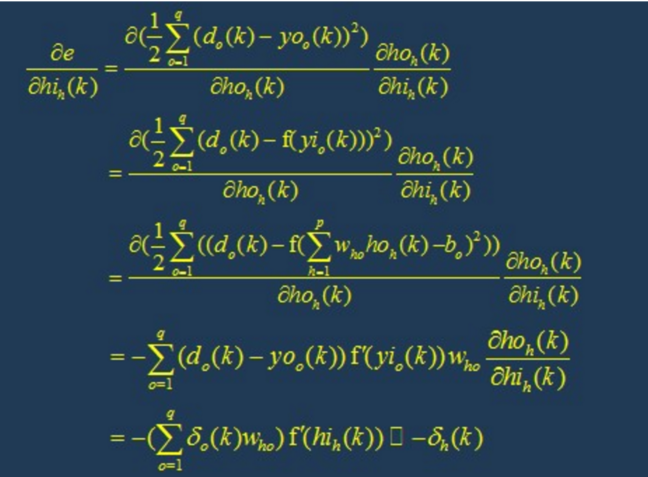第六步：修正权值
利用输出层各神经元的

δ

o

(

k

)

\delta_o(k)

和隐含层各神经元的输出来修正连接权值

w

h

o

(

k

)

w_{ho}(k)

。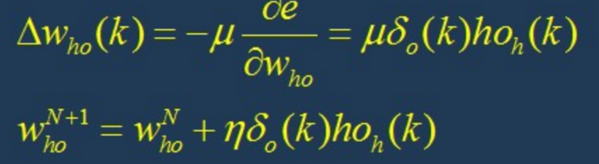第七部：修正权值
利用隐含层各神经元的

δ

h

(

k

)

\delta_h(k)

和输入层各神经元的输入修正连接权值。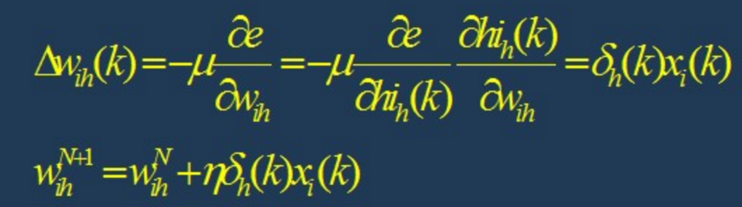第八步：计算全局误差

E

=

1

2

m

∑

k

=

1

m

∑

o

=

1

q

(

d

o

(

k

)

−

y

o

(

k

)

)

2

E={1\over 2m}\sum_{k=1}^m\sum_{o=1}^q(d_o(k)-y_o(k))^2

第九步：判断模型合理性
判断网络误差是否满足要求。 当误差达到预设精度或者学习次数大于设计的最大次数，则结束算法。 否则，选取下一个学习样本以及对应的输出期望，返回第三部，进入下一轮学习。
BP网络的设计
在进行BP网络的设计是，一般应从网络的层数、每层中的神经元个数和激活函数、初始值以及学习速率等几个方面来进行考虑，下面是一些选取的原则。
1.网络的层数
理论已经证明，具有偏差和至少一个S型隐层加上一个线性输出层的网络，能够逼近任何有理函数，增加层数可以进一步降低误差，提高精度，但同时也是网络 复杂化。另外不能用仅具有非线性激活函数的单层网络来解决问题，因为能用单层网络解决的问题，用自适应线性网络也一定能解决，而且自适应线性网络的 运算速度更快，而对于只能用非线性函数解决的问题，单层精度又不够高，也只有增加层数才能达到期望的结果。
2.隐层神经元的个数
网络训练精度的提高，可以通过采用一个隐含层，而增加其神经元个数的方法来获得，这在结构实现上要比增加网络层数简单得多。一般而言，我们用精度和 训练网络的时间来恒量一个神经网络设计的好坏： （1）神经元数太少时，网络不能很好的学习，训练迭代的次数也比较多，训练精度也不高。 （2）神经元数太多时，网络的功能越强大，精确度也更高，训练迭代的次数也大，可能会出现过拟合(over fitting)现象。 由此，我们得到神经网络隐层神经元个数的选取原则是：在能够解决问题的前提下，再加上一两个神经元，以加快误差下降速度即可。
3.初始权值的选取
一般初始权值是取值在(−1,1)之间的随机数。另外威得罗等人在分析了两层网络是如何对一个函数进行训练后，提出选择初始权值量级为s√r的策略， 其中r为输入个数，s为第一层神经元个数。
4.学习速率
学习速率一般选取为0.01−0.8，大的学习速率可能导致系统的不稳定，但小的学习速率导致收敛太慢，需要较长的训练时间。对于较复杂的网络， 在误差曲面的不同位置可能需要不同的学习速率，为了减少寻找学习速率的训练次数及时间，比较合适的方法是采用变化的自适应学习速率，使网络在 不同的阶段设置不同大小的学习速率。
5.期望误差的选取
在设计网络的过程中，期望误差值也应当通过对比训练后确定一个合适的值，这个合适的值是相对于所需要的隐层节点数来确定的。一般情况下，可以同时对两个不同 的期望误差值的网络进行训练，最后通过综合因素来确定其中一个网络。
BP网络的局限性
BP网络具有以下的几个问题：
(1)需要较长的训练时间：这主要是由于学习速率太小所造成的，可采用变化的或自适应的学习速率来加以改进。(2)完全不能训练：这主要表现在网络的麻痹上，通常为了避免这种情况的产生，一是选取较小的初始权值，而是采用较小的学习速率。(3)局部最小值：这里采用的梯度下降法可能收敛到局部最小值，采用多层网络或较多的神经元，有可能得到更好的结果。 BP网络的改进

P算法改进的主要目标是加快训练速度，避免陷入局部极小值等，常见的改进方法有带动量因子算法、自适应学习速率、变化的学习速率以及作用函数后缩法等。 动量因子法的基本思想是在反向传播的基础上，在每一个权值的变化上加上一项正比于前次权值变化的值，并根据反向传播法来产生新的权值变化。而自适应学习 速率的方法则是针对一些特定的问题的。改变学习速率的方法的原则是，若连续几次迭代中，若目标函数对某个权倒数的符号相同，则这个权的学习速率增加， 反之若符号相反则减小它的学习速率。而作用函数后缩法则是将作用函数进行平移，即加上一个常数。
BP网络实现
由于BP网络具有出色的非线性映射能力、泛化能力和容错能力，因此BP网络成了至今为止应用最广泛的人工神经网络。下图是Matlab下用BP网络做线性拟合的结果，效果很好。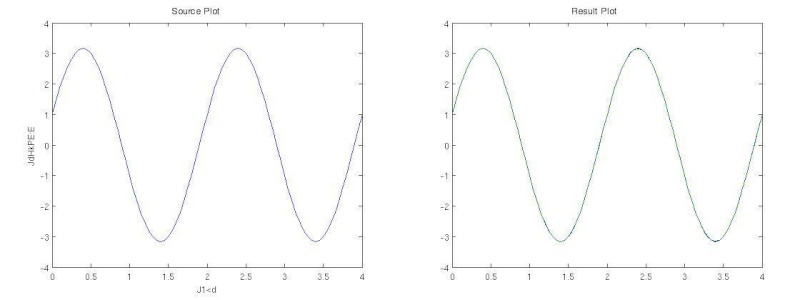% BP网络函数逼近实例
% 1.首先定义正弦函数，采样率为20Hz，频率为1Hz
k = 1; % 设定正弦信号频率
p = [0:0.05:4];
t = cos(k*pi*p) + 3*sin(pi*p);
plot(p, t, '-'), xlabel('时间'); ylabel('输入信号');
% 2.生成BP网络。用newff函数生成前向型BP网络，设定隐层中神经元数目为10
% 分别选择隐层的传递函数为 tansig，输出层的传递函数为 purelin，
% 学习算法为trainlm。
net =
newff(minmax(p),[10,10,1],{'tansig','tansig','purelin'},'trainlm');
% 3.对生成的网络进行仿真并做图显示。
y1 = sim(net,p); plot(p, t, '-', p, y1, '--')
% 4.训练。对网络进行训练，设定训练误差目标为 1e-5，最大迭代次数为300，
% 学习速率为0.05。
net.trainParam.lr=0.05;
net.trainParam.epochs=1000;
net.trainParam.goal=1e-5;
[net,tr]=train(net,p,t);
%5.再次对生成的网络进行仿真并做图显示。
y2 = sim(net,p);
plot(p, t, '-', p, y2, '--')

这是用C语言写的：用BP神经网络拟合函数：

Y

=

s

i

n

(

X

)

Y=sin(X)

#include "math.h"
#include "time.h"
#include "stdio.h"
#include "stdlib.h"
#include "ctype.h"
#define Ni 1
#define Nm 4
#define No 1
#define L 100
#define Enom 0.02
#define loopmax 100000
#define e 2.71828
double E;
double a,u,n;
double W1[Ni][Nm],D1[Ni][Nm],W2[Nm][No],D2[Nm][No];
double D22[Nm][No],D11[Ni][No];
double a1[Ni][Nm],a2[Nm][No];
double Pi[L][Ni],Pm[L][Nm],Po[L][No],T[L][No];
double Xm[L][Nm],Xo[L][No];
double Qm[L][Nm],Qo[L][No];
void proceed();
void proceedR();
void forQ();
void amend();
void initiate();
double newa(double a,double D);
double cal(double d);
double vcal(double d);
main()
{
long int i;
int flag;
char choice;
for(;;)
{
flag=0;
initiate();
for(i=0;;i++)
{
proceed();
if( E < Enom )
{
flag=1;
break;
}
if( i >= loopmax)
{
flag = -1;
break;
}
if(i%2500==0)
printf("第%10d轮误差：%20f,学习速率:%10f\n",i,E,a1);
forQ();
amend();
}
if(flag>0)proceedR();
else printf("训练失败！\n");
for(;;)
{
choice=getchar();
printf("是否继续？(Y/N)\n");
choice=getchar();
choice=toupper(choice);
if(choice=='Y')break;
if(choice=='N')exit(0);
}
}
}
void initiate()
{
int i,j;
int random;
double x;
double step;
int stime;
long ltime;
ltime=time(NULL);
stime=(unsigned)ltime/2;
srand(stime);
a=0.02;
u=1;
n=1;
printf("本程序将用BP神经网络拟合函数：Y=sin(X)\n\n");
for( i=0; i<Nm; i++)
{
for( j=0; j<Ni; j++)
{
random=rand()%100-50;
x=random;
x=x/100;
W1[j][i]=x;
D11[j][i]=0;
D1[j][i]=0;
a1[j][i]=0.01;
}
for( j=0; j<No; j++)
{
random=rand()%100-50;
x=random;
x=x/100;
W2[i][j]=x;
D22[i][j]=0;
D2[i][j]=0;
a2[i][j]=0.01;
}
}
step=1.0/L;
for(i=0;i<L;i++)
{
x=i;
Pi[i]=x*step;
T[i]=sin(Pi[i]);
}
printf("初始化成功!\n\n下面将对神经网络进行训练请稍候。\n");
}
void proceed()
{
int i, j, k;
E=0 ;
for( i=0; i<L; i++ )
{
for( j=0; j<Nm; j++ )
{
Pm[i][j] = 0;
for( k=0; k<Ni; k++ )
{
Pm[i][j] = Pi[i][k] * W1[k][j] + Pm[i][j];
}
Xm[i][j] = cal( Pm[i][j] );
}
for( j=0; j<No; j++)
{
Po[i][j] = 0;
for( k=0; k<Nm; k++)
{
Po[i][j] = Xm[i][k] * W2[k][j] + Po[i][j];
}
Xo[i][j] = cal( Po[i][j] );
E = E + ( Xo[i][j] - T[i][j] ) * ( Xo[i][j] - T[i][j] ) / 2;
}
}
}
void forQ()
{
int i,j,k;
for( i=0; i<L; i++ )
{
for( j=0; j<No; j++)
{
Qo[i][j] = ( T[i][j] - Xo[i][j] )* vcal( Xo[i][j] );
}
for(j=0; j<Nm; j++)
{
Qm[i][j]=0;
for( k=0; k<No; k++)
{
Qm[i][j] = Qo[i][k] * W2[j][k] + Qm[i][j];
}
Qm[i][j] = Qm[i][j] * vcal( Xm[i][j] );
}
}
}
void amend()
{
int i,j,k;
double D;
for( i=0; i<Nm; i++)
{
for( j=0; j<Ni; j++)
{
D1[j][i]=0;
}
for( j=0; j<No; j++)
{
D2[i][j]=0;
}
}
for( i=0; i<Ni; i++)
{
for( j=0; j<Nm; j++)
{
for( k=0; k<L; k++)
{
D1[i][j] = Qm[k][j] * Pi[k][i] + D1[i][j];
}
D = D1[i][j] * D11[i][j]  ;//为D11付初值
a1[i][j] = newa( a1[i][j] , D );  // a 付初值
W1[i][j] = W1[i][j] + a1[i][j] * ( n * D1[i][j] + ( 1 - n ) * D11[i][j] );
D11[i][j] = D1[i][j];
}
}
for( i=0; i<Nm; i++)
{
for( j=0; j<No; j++)
{
for( k=0; k<L; k++)
{
D2[i][j] = Qo[k][j] * Xm[k][i] + D2[i][j];
}
D = D2[i][j] * D22[i][j]  ;//为D11付初值
a2[i][j] = newa( a2[i][j] , D );
W2[i][j] = W2[i][j] + a2[i][j] * ( n * D2[i][j] + ( 1 - n ) * D22[i][j] );
D22[i][j] = D2[i][j];
}
}
}
void proceedR()
{
int i, j;
float x;
double input,output;
char choice;
for(;;)
{
for(;;)
{
printf("在此输入需要计算的值(0,1):\n");
scanf("%f",&x);
input=(double)x;
if((input>=0)&(input<=1))break;
printf("注意输入值应介于0、1之间！\n");
for(;;)
{
choice=getchar();
printf("是否继续？(Y/N)\n");
choice=getchar();
choice=toupper(choice);
if(choice=='Y')break;
if(choice=='N')exit(0);
}
}
for(i=0;i<Nm;i++)
{
Pm[i]=0;
for( j=0; j<Ni; j++ )
{
Pm[i] =  input* W1[j][i]+Pm[i] ;
}
Xm[i] = cal( Pm[i] );
}
for( i=0; i<No; i++)
{
Po[i] = 0;
for( j=0; j<Nm; j++)
{
Po[i] = Xm[j] * W2[j][i]+Po[i];
}
}
output=cal( Po );
printf("输入值为%20f对应的结果为%f\n",input,output);
printf("输入值为%20f对应的正常结果为%f\n",input,sin(input));
for(;;)
{
choice=getchar();
printf("是否继续？(Y/N)\n");
choice=getchar();
choice=toupper(choice);
if(choice=='Y')break;
if(choice=='N')exit(0);
}
}
}

double newa(double a, double D)
{
if( D > 0 )
{
{
if(a<=0.04)
a = a * 2;
else a=0.08;
}
}
else
if ( D < 0)
{
if(a>=0.02)
{
a = a / 2;
}
else a=0.01;
}
return a;
}
double cal(double d)
{
d =  - (d * u);                                //              chushihua
d = exp( d );
d = 1 / ( 1 + d );
return d;
}
double vcal(double d)
{
return u * d * ( 1 - d );
}


展开全文• BP 神经网络算法识别MNIST手写数字数据集 人工神经网络的基本原理 生物神经元在结构上由: 细胞体(Cell body) 树突(Dendrite) 轴突(Axon) 突触(Synapse) 四部分组成。用来完成神经元间信息的接收、传递和处理。 ...
BP 神经网络算法识别MNIST手写数字数据集
人工神经网络的基本原理
生物神经元在结构上由:
细胞体(Cell body)树突(Dendrite)轴突(Axon)突触(Synapse)
四部分组成。用来完成神经元间信息的接收、传递和处理。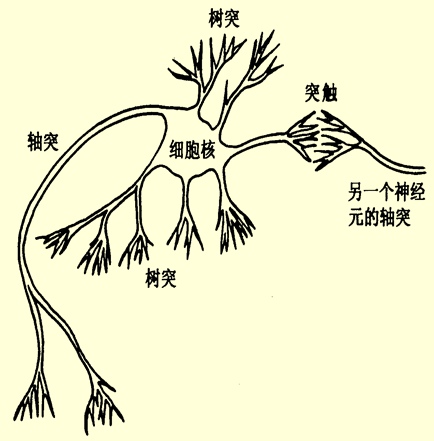神经元及其突触是神经网络的基本器件。因此，模拟生物神经网络应首先模拟生物神经元, 人工神经元(节点)从三个方面进行模拟:
节点本身的信息处理能力节点与节点之间连接(拓扑结构)相互连接的强度(通过学习来调整)
神经元模型
一般地，将形式神经元模型表示为下图所示的一个具有多输入-单输出的非线性阈值器件。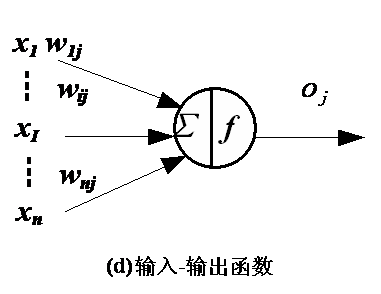x

1

,

x

2

,

…

,

x

n

x_1, x_2, \dots, x_n

表示某一神经元的第n个输入;

W

i

j

W_{ij}

表示第

i

i

个神经元与第

j

j

个神经元的突触连接强度，其称为权值。神经元模型将这些输入加权后经激励函数

f

f

输出。

A

j

=

∑

j

=

1

n

x

i

W

i

j

−

Θ

j

A_j = \sum_{j=1}^nx_iW_{ij} - \Theta_j

O

j

=

f

(

A

j

)

O_j = f(A_j)

几个常见的神经元模型
阈值型 该类型的神经元具有一个设定的阈值，是一种最简单的神经元，当加权后的输入大于阈值时，输出为1，否则为0。 激励函数一般表示为：

y

i

=

f

(

A

i

)

=

{

1

,

A

i

&gt;

Θ

i

0

,

A

i

≤

Θ

i

y_i = f(A_i) = \begin{cases} 1, \quad A_i \gt \Theta_i \\\\ 0, \quad A_i \leq \Theta_i \end{cases}

对于阈值型神经元, 其输入权值

W

i

j

W_{ij}

可在

(

−

1

,

+

1

)

(-1, +1)

区间连续取值。负值表示抑制，正值表示加强。线性饱和型 线性饱和型神经元在一定区间内，输入输出满足线性关系。激励函数为：

y

i

=

f

(

A

i

)

=

{

0

,

A

i

&lt;

0

C

A

i

,

0

≤

A

i

≤

A

c

1

,

A

i

&gt;

A

c

y_i = f(A_i) = \begin{cases} 0, \quad A_i \lt 0 \\\\ CA_i, \quad 0 \leq A_i \leq A_c \\\\ 1, \quad A_i \gt A_c \end{cases}

C

C

为常量S(sigmoid)型 S型神经元是一类连续型神经元模型，采用S型函数(sigmoid 函数)。其激励函数为：

y

i

=

f

(

A

i

)

=

1

/

(

1

+

e

A

i

)

y_i = f(A_i) = 1/(1+ e^{A_i})

或

y

i

=

f

(

A

i

)

=

1

/

(

2

(

1

+

t

h

(

A

i

/

A

0

)

)

)

y_i = f(A_i) = 1/(2(1+ th(A_i/A_0)))

子阈累积型 这类神经元的激活函数也是一个非线性的。当所产生的激活值超过

T

T

值时，该神经元将被激活。在线性范围内，系统反响是线性的。子阈的一个作用是抑制噪声，即对小的随机输入不产生响应。概率型 其输出状态的0或1将根据激活函数的大小按照一定的概率来确定。 假定神经元为1的概率为：

P

(

S

i

=

1

)

=

1

/

(

1

+

e

A

i

T

)

P(S_i = 1) = 1 / (1 + e^{\frac{A_i}{T}})

则，状态为0的概率为:

P

(

S

i

=

0

)

=

1

−

P

(

S

i

=

1

)

P(S_i = 0) = 1 - P(S_i = 1)

玻尔兹曼神经元就是这一类神经元。
多层前向神经网络及BP学习算法
单层前向神经网络的缺点是只能解决线性可分的分类问题，要增强网络的分类能力唯一的方法是采用多层网络。也就是在输入层和输出层之间加入隐层，这就构成了多层感知机。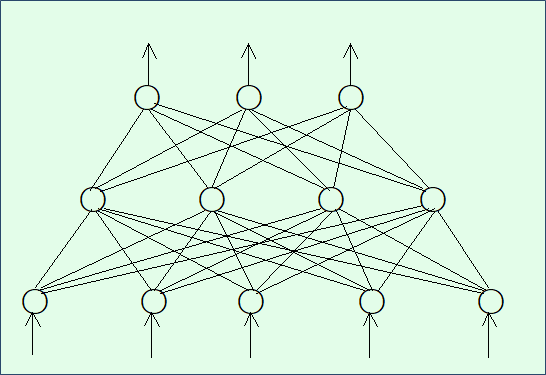BP学习算法
在BP学习算法中：
工作信号正向传播;误差信号反向传播。
BP算法的具体步骤如下(三层的BP学习算法)：
设置变量和参量:
输入数据将被作为一个

n

n

维的输入向量

x

k

=

[

x

k

1

,

x

k

2

,

…

,

x

k

N

]

(

k

=

1

,

2

,

…

,

N

)

x_k = [x_{k1}, x_{k2}, \dots, x_{kN}] \quad (k = 1, 2, \dots, N)

或称训练样本;

N

N

为训练样本的个数。设：

W

I

H

(

n

)

=

[

w

11

(

n

)

w

12

(

n

)

…

w

1

H

(

n

)

w

21

(

n

)

w

22

(

n

)

…

w

2

H

(

n

)

⋮

⋮

⋱

⋮

w

I

1

(

n

)

w

I

2

(

n

)

…

w

I

H

(

n

)

]

W_{IH}(n) = \begin{bmatrix} w_{11}(n) &amp; w_{12}(n) &amp; \ldots &amp; w_{1H}(n) \\\\ w_{21}(n) &amp; w_{22}(n) &amp; \ldots &amp; w_{2H}(n) \\\\ \vdots &amp; \vdots &amp; \ddots &amp; \vdots \\\\ w_{I1}(n) &amp; w_{I2}(n) &amp; \ldots &amp; w_{IH}(n) \end{bmatrix}

为第

n

n

次迭代时,输入层和隐层之间的权值矩阵。

W

H

O

(

n

)

=

[

w

11

(

n

)

w

12

(

n

)

…

w

1

O

(

n

)

w

21

(

n

)

w

22

(

n

)

…

w

2

O

(

n

)

⋮

⋮

⋱

⋮

w

H

1

(

n

)

w

H

2

(

n

)

…

w

H

O

(

n

)

]

W_{HO}(n) = \begin{bmatrix} w_{11}(n) &amp; w_{12}(n) &amp; \ldots &amp; w_{1O}(n) \\\\ w_{21}(n) &amp; w_{22}(n) &amp; \ldots &amp; w_{2O}(n) \\\\ \vdots &amp; \vdots &amp; \ddots &amp; \vdots \\\\ w_{H1}(n) &amp; w_{H2}(n) &amp; \ldots &amp; w_{HO}(n) \end{bmatrix}

为第

n

n

次迭代时,隐层和输出层之间的权值矩阵。

Y

k

(

n

)

=

[

y

k

1

(

n

)

,

y

k

2

(

n

)

,

…

,

y

k

N

(

n

)

]

(

k

=

1

,

2

,

…

,

N

)

Y_k(n) = [y_{k1}(n), y_{k2}(n), \dots ,y_{kN}(n)] \quad (k = 1, 2, \dots, N)

为第

n

n

次迭代时网络的实际输出

d

k

(

n

)

=

[

d

k

1

(

n

)

,

d

k

2

(

n

)

,

…

,

d

k

N

(

n

)

]

(

k

=

1

,

2

,

…

,

N

)

d_k(n) = [d_{k1}(n), d_{k2}(n), \dots ,d_{kN}(n)] \quad (k = 1, 2, \dots, N)

为第

n

n

次迭代时网络的期望输出。由于在本次实验中使用的是S型神经元,其特性不能达到渐进值

+

α

+\alpha

和

−

α

-\alpha

, 故期望输出应当取接近渐进值的值，而不是取

+

α

+\alpha

和

−

α

-\alpha

。

η

\eta

为学习效率,

n

n

为迭代次数。 初始化，赋给

W

I

H

(

0

)

,

W

H

O

(

0

)

W_{IH}(0), W_{HO}(0)

各一个较小的随机非零值，建议初始值分布在区间

(

−

2.4

/

F

,

2.4

/

F

)

(-2.4/F, 2.4/F)

或

(

−

3

/

F

,

3

/

F

)

(-3/\sqrt{F}, 3/\sqrt{F})

之间。

F

F

为所连单元的输入端个数。输入随机样本

x

k

x_k

,并置

n

=

0

n=0

对输入样本

x

k

x_k

,前向计算BP神经网络每层的输入信号

u

u

和输出信号

v

v

。其中

v

o

O

(

n

)

=

y

k

o

(

n

)

o

=

1

,

2

,

…

,

O

v_o^O(n) = y_{ko}(n) \quad o = 1, 2 , \dots, O

由期望输出

d

k

d_k

和上一步求得的实际输出

Y

k

(

n

)

Y_k(n)

计算误差

E

(

n

)

E(n)

判断其是否满足要求，如满足要求转至第8步。判断

n

+

1

n+1

是否大于最大迭代次数，若大于转至第8步，否则对输入样本

x

k

x_k

反向计算每层神经元的局部梯度

δ

\delta

。其中:

δ

o

O

(

n

)

=

y

o

(

n

)

(

1

−

y

o

(

n

)

)

(

d

o

(

n

)

−

y

o

(

n

)

)

o

=

1

,

2

,

…

,

O

\delta_o^O(n) = y_o(n)(1-y_o(n))(d_o(n) - y_o(n)) \quad o= 1, 2, \dots, O

δ

h

H

(

n

)

=

f

′

(

u

h

H

(

n

)

)

∑

h

=

1

H

δ

o

O

(

n

)

w

h

o

(

n

)

h

=

1

,

2

,

…

,

H

\delta_h^H(n) = f&#x27;(u_h^H(n))\sum_{h=1}^H\delta_o^O(n)w_{ho}(n) \quad h= 1, 2, \dots, H

按下式计算权值修正值

Δ

w

\Delta w

,并修正权值;

n

=

n

+

1

n = n + 1

, 转至第4步:

Δ

w

h

o

(

n

)

=

η

δ

o

O

(

n

)

v

h

H

w

h

o

(

n

+

1

)

=

w

h

o

(

n

)

+

Δ

w

h

o

(

n

)

\Delta w_{ho}(n) = \eta\delta_o^O(n)v_h^H \quad w_{ho}(n+1) = w_{ho}(n) + \Delta w_{ho}(n)

Δ

w

i

h

(

n

)

=

η

δ

h

H

(

n

)

v

i

I

w

i

h

(

n

+

1

)

=

w

i

h

(

n

)

+

Δ

w

i

h

(

n

)

\Delta w_{ih}(n) = \eta\delta_h^H(n)v_i^I \quad w_{ih}(n+1) = w_{ih}(n) + \Delta w_{ih}(n)

判断是否学完所有的训练样本，若是则结束，否则转回第3步。
注:
对于一个训练周期训练多个样本的的训练模式，修正值的计算函数方法如下:

E

a

v

=

1

N

∑

k

=

1

N

E

k

=

1

2

N

∑

k

=

1

N

∑

p

=

1

P

e

k

p

2

E_{av} = \frac{1}{N}\sum_{k=1}^NE_k = \frac{1}{2N}\sum_{k=1}^N\sum_{p=1}^Pe_{kp}^2

其中，

e

k

p

2

e_{kp}^2

为网络输入第

k

k

个训练样本时输出神经元

p

p

的误差。
学习步长

η

\eta

值的选取比较重要，

η

\eta

值过大会引起网络震荡，过小则会导致收敛速度太慢
隐层个数的选取有两种：
大于

log

⁡

2

F

\log_2 F

,

F

F

为该层的输入节点个数

I

+

O

+

a

\sqrt{I+O} + a

其中,

a

a

为一个

1

−

10

1-10

的修正值，

I

,

O

I, O

分别为输入层和输出层的节点个数
BP学习算法并不完善，它主要存在一下缺陷：学习收敛速度太慢，网络的学习记忆具有不稳定性。
python实现
class BPNet:
def train(self):
labels = []
with open(train_labels_idx1_ubyte_file, "rb") as file:
if magicNumber == 2049:
print("label")
else :
print("Can't recognized")
return 0
with open(train_images_idx3_ubyte_file, "rb") as file:
magicNumber, numOfImages, rowsNum, columnsNum = struct.unpack('>iiii', file.read(16))
if magicNumber == 2051:
print("image")
else :
print("Can't recognized")
return 0
# 神经元节点
# 输入层, 隐层, 输出层
inputLayerNodeNum, hideLayerNodeNum, outputLayerNodeNum = rowsNum * columnsNum, 120, 10
# 权值
self.weightIH, self.weightHO = [[] for i in range(inputLayerNodeNum)], [[] for i in range(hideLayerNodeNum)]
self.weightGenerator(self.weightIH, inputLayerNodeNum, hideLayerNodeNum)
self.weightIH = np.array(self.weightIH)
self.weightGenerator(self.weightHO, hideLayerNodeNum, outputLayerNodeNum)
self.weightHO = np.array(self.weightHO)
count, formatStr, yita = 0, '>' + str(inputLayerNodeNum) + 'B', 0.1
while count < numOfImages:
Ioutput = 1 / (1 + np.exp(-1 * pixes))
Houtput = self.f(Ioutput, self.weightIH)
Ooutput = self.f(Houtput, self.weightHO)
EO = Ooutput * (1 - Ooutput) * (self.d(labels[count]) - Ooutput)
EH = Houtput * (1 - Houtput) * (self.weightHO @ EO.T).T
self.weightHO = self.weightHO + yita * Houtput.T @ EO
self.weightIH = self.weightIH + yita * Ioutput.T @ EH
count = count + 1
if np.mod(count, 1000) == 0:
print("已训练 " + str(count) + " 张图片")
self.test()

展开全文人工智能 机器学习 python
• 数据集大小3*3000，代表3000个二维样本，第三行为输出
• 这篇文章我们一起学习一下如何使用PyTorch搭建神经网络训练分类模型，这里我们用的数据集是Yann LeCun的MINIST数据集。首先我们来看看需要哪些python库： import torch import torch.nn as nn import torch.nn....PyTorch 分类
• 基于Tensorflow针对cifar数据集运用卷积神经网络解决100类图片的分类问题。tensorflow 图像识别
• python实现RBF神经网络识别Mnist数据集 作者：ExcelMann，转载需注明。 话不多说，直接贴代码，代码有注释。 # Author:Xuangan, Xu # Data:2020-11-11 """ RBF神经网络 ----------------- 设计RBF神经网络实现手写...python 深度学习 网络
• 在这节的内容中，我们用之前讲述的内容来搭建一个比较简单的卷积神经网络，实现MNIST手写数字体数据集的图片分类。 我们给出本次实验的CNN网络参数配置如下： 原始数据：输入为[28,28]，输出为[1,10] 卷积核1：[5,5]...卷积 机器学习 深度学习
• python实现BP神经网络识别Mnist数据集 作者：ExcelMann，转载需注明。 话不多说，直接贴代码，代码有注释。 # Author:Xuangan, Xu # Data:2020-10-28 """ BP神经网络 ----------------- 利用梯度下降法，实现MNIST...python 深度学习 机器学习
• 前言：经过将近一个月的陆续学习研究，在神经网络中训练多分类任务识别Cityscapes数据集，终于在最近得到了理想中的实验结果。在我陷入对细节参数调整不当及诸多问题时，苦于没有一篇能够“面向新手编程”的博客，...深度学习 自动驾驶 tensorflow
• 这里是**手势识别 雷达信号处理与卷积神经网络 （1）**，教你如何制作自己的数据集。 ” 我们经常使用开源数据集训练网络，那么当遇到特定的问题，没有合适的开源数据集时，如何获取并制作自己的数据集呢？手势识别 雷达信号处理 创建
• 基于python的BP神经网络算法对mnist数据集的识别 目录： 1. mnist数据集 1.1 mnist数据集是什么 1.2 mnist数据集的读取** 2. 神经网络 2.1 批处理数据 2.2 前向传播 2.2.1 sigmoid和softmax函数 2.2.2 损失函数 ...深度学习 python 矩阵
• ## 卷积神经网络

万次阅读 多人点赞 2014-11-29 16:20:41
自今年七月份以来，一直在实验室负责卷积神经网络（Convolutional Neural Network，CNN），期间配置和使用过theano和cuda-convnet、cuda-convnet2。为了增进CNN的理解和使用，特写此博文，以其与人交流，互有增益。...
• 基于卷积神经网络的高光谱分类 一、研究现状 只考虑到高光谱图像的光谱特征，即：1、提取特征(小波变换、Gabor纹理分析、形态学剖面) ​ 2、利用分类方法分类(支持向量机、决策树、随机森林、神经...算法 python
• 本文参考了经典的LeNet-5卷积神经网络模型对mnist数据集进行训练。LeNet-5模型是大神Yann LeCun于1998年在论文"Gradient-based learning applied to document recognition"中提出来的，它是第一个成功应用...深度学习 tensorflow mnist
• 本文针对mnist手写数字，搭建了四层简单的神经网络进行图片的分类，详细心得记录下来分享 我是采用的TensorFlow框架进行的训练 import tensorflow as tf from tensorflow.examples.tutorials.mnist import input...
• 1.CIFAR-10数据集介绍 本节使用的是比较经典的数据集叫CIFAR-10,包含60000张32*32的彩色图像（总算不像MNIST，是灰度图了，灰度图是单通道），因为是彩色图像，所以这个数据集是三通道的，分别是R,G,B三个通道。...tensorflow
• 将五种花朵图片转化为TFRecord文件数据集，进行卷积神经网络训练，网络架构为：卷积层×2+池化层×2+全连接神经网络层。TensorFlow
• MachineLP的Github（欢迎follow）：https://github.com/MachineLP本小节使用torch搭建线性...（2）定义训练数据，加餐部分是使用自己的数据集：（可参考：https://blog.csdn.net/u014365862/article/details/80506...torch
• 神经网络学习小记录19——微调VGG分类模型训练自己的数据（猫狗数据集）学习前言什么是VGG16模型VGG模型的复杂程度训练前准备1、数据集处理2、创建Keras的VGG模型3、下载VGG16的权重开始训练1、训练的主函数2、全部...微调 VGG 分类
• 本文主要介绍近年来图神经网络方向使用最多的三个数据集的详细内容：Cora、Citeseer、PubMed。 Items Cora Citeseer PubMed #Nodes 2708 3327 19717 #Edges 5429 4732 44338 #...
• 0. MNIST：BP: 98%1. Norb：BP: 88%左右2. cifar-10:BP: 50%《Understanding the difficulty of training deep feedforward neural networks》3. fashion-MNIST：其他：常用机器学习数据集精度
• 神经网络学习小记录17——使用AlexNet分类模型训练自己的数据（猫狗数据集）学习前言什么是AlexNet模型训练前准备1、数据集处理2、创建Keras的AlexNet模型开始训练1、训练的主函数2、Keras数据生成器3、主训练函数...AlexNet 分类
• 因为CNN卷积神经网络用MNIST数据集、sklearn数据集代码很多部分都很相似，这一篇就不附详细说明，源码如下。CNN卷积神经网络的工作原理，请详情参考——【深度学习】5：CNN卷积神经网络原理、MNIST数据集识别 –—-...sklearn 数字识别
• 1、神经网络模型 2、明确任务以及参数 1）待估参数： 2）超参数： 3）任务 3、神经网络数学模型定义 1）激活函数 ​ 2）各层权重、阈值定义 3）各层输入输出定义 4、优化问题的目标函数与迭代公式 1）...算法 python 机器学习 深度学习
• 一、实验内容 ...本次实验目的是用Bp神经网络对iris数据集进行分类，并进行分析。 二、实验过程 1.将数据集加载到工作区：load iris.dat; 2.数据预处理 （1）将原始iris数据集随机抽取118行作为训练数...人工智能 模式识别 分类器
• 有半个多月没有碰神经网络了，因为之前做的一个去噪声的效果很不好，有点打击信心。于是把注意力集中在计算成像方面，但最近也是遇到了瓶颈，发现编码孔径成像理论似乎已经挖的差不多了，毕竟上世纪六七十年代就已经...
• IDE：pycharm Python: Python3.6 OS: win10 tf : CPU版本 ...一、CIFAR10数据集 数据集代码下载 from tensorflow.models.tutorials.image.cifar10 import cifar10 cifar10.maybe_dow...python...